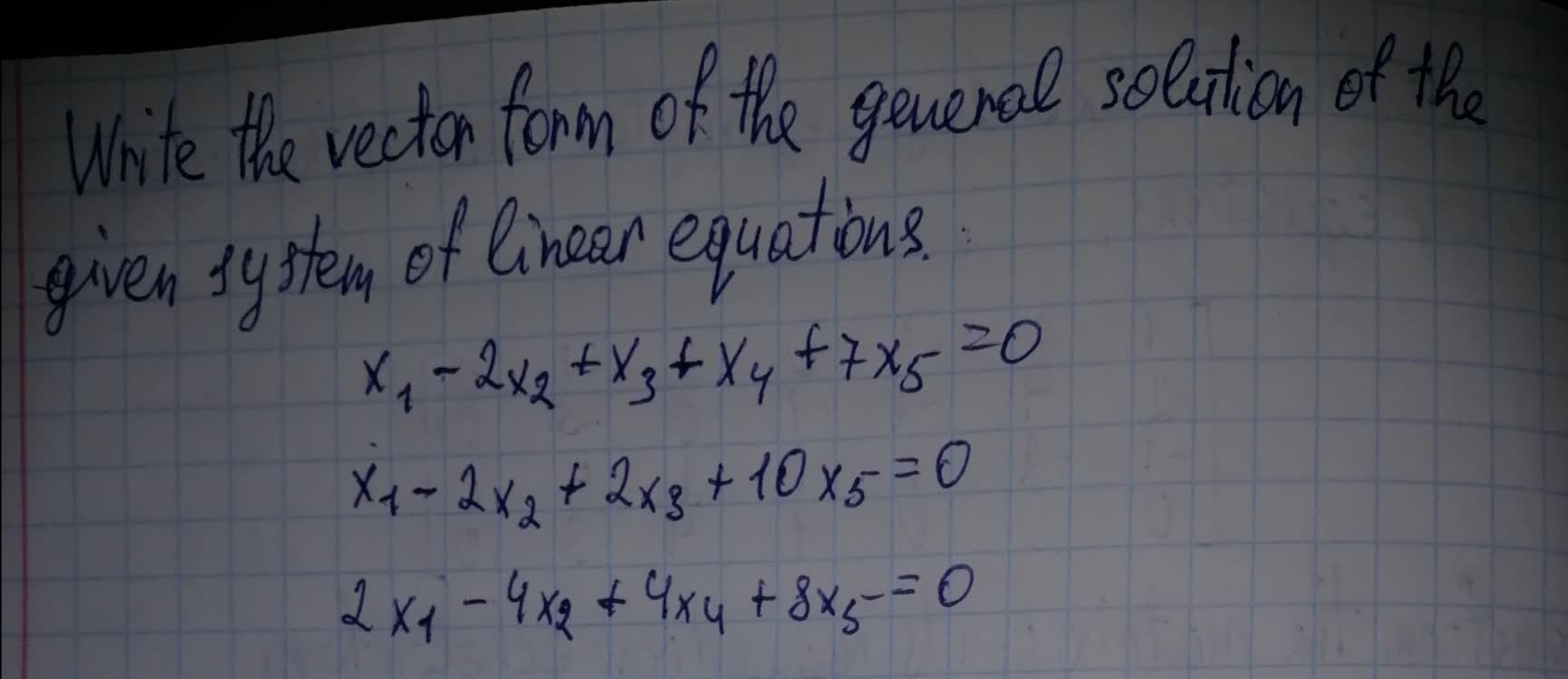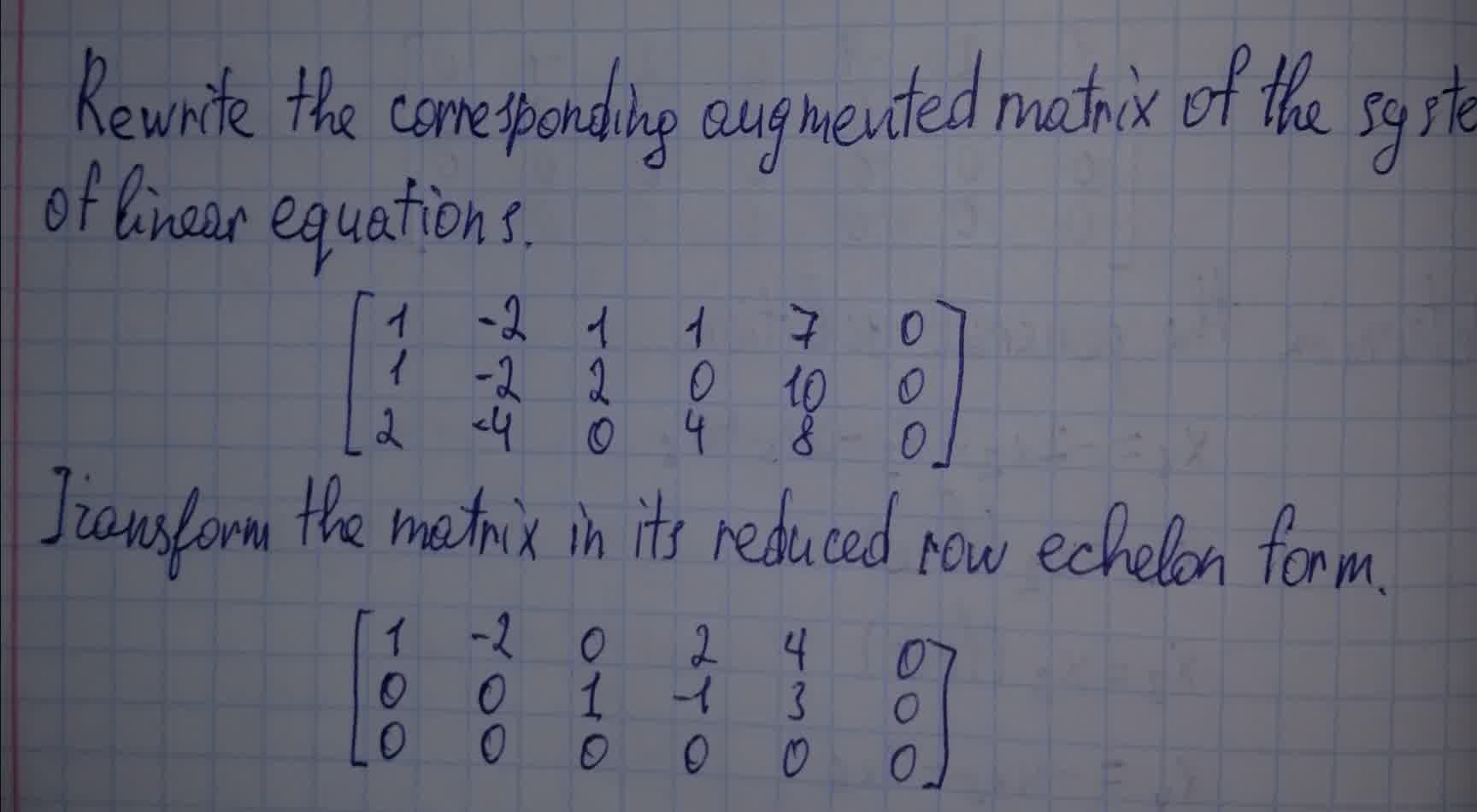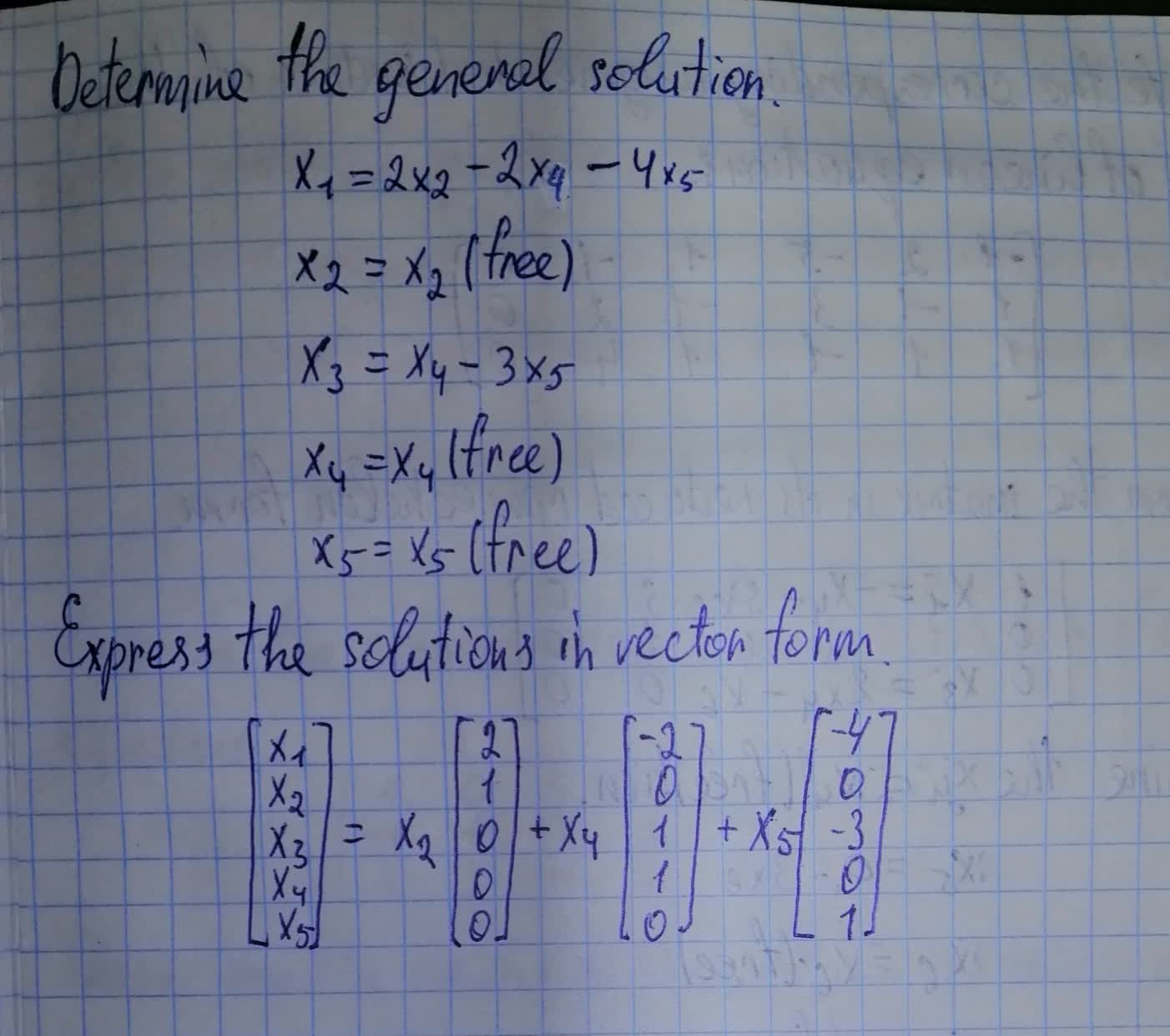# Write the vector form of the general solution of the given system of linear equations. x_1-2x_2+x_3+x_4+7x_5=0Dottie Parra 2021-08-06 Answered
Write the vector form of the general solution of the given system of linear equations. ${x}_{1}-2{x}_{2}+{x}_{3}+{x}_{4}+7{x}_{5}=0$You can still ask an expert for help

• Questions are typically answered in as fast as 30 minutes

Solve your problem for the price of one coffee

• Math expert for every subject
• Pay only if we can solve itBenedict

Vector form looks like this:###### Not exactly what you’re looking for?Vasquez

Rewrite the corresponding augmented matrix of the system of linear equation

$\left[\begin{array}{cccccc}1& -2& 1& 1& 7& 0\\ 1& -2& 2& 0& 10& 0\\ 2& -4& 0& 4& 8& 0\end{array}\right]$

Transform the matrix in its reduced row echelon form

$\left[\begin{array}{cccccc}1& -2& 0& 2& 4& 0\\ 0& 0& 1& -1& 3& 0\\ 0& 0& 0& 0& 0& 0\end{array}\right]$

Determine the general solution

${x}_{1}=2{x}_{2}-2{x}_{4}-4{x}_{5}$
${x}_{2}={x}_{2}$ (free)
${x}_{3}={x}_{4}-3{x}_{5}$
${x}_{4}={x}_{4}$
${x}_{5}={x}_{5}$ (free)

Express the solutions in vector form

$\left[\begin{array}{c}{x}_{1}\\ {x}_{2}\\ {x}_{3}\\ {x}_{4}\\ {x}_{5}\end{array}\right]={x}_{2}\left[\begin{array}{c}2\\ 1\\ 0\\ 0\\ 0\end{array}\right]+{x}_{4}\left[\begin{array}{c}-2\\ 0\\ 1\\ 1\\ 0\end{array}\right]+{x}_{5}\left[\begin{array}{c}-4\\ 0\\ -3\\ 0\\ 1\end{array}\right]$